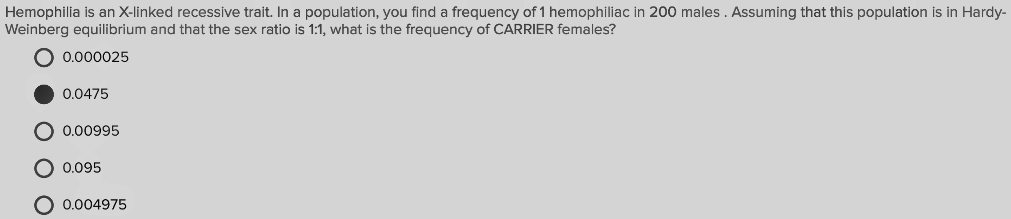# Problem: Hemophilia is an X-linked recessive trait. In a population, you find a frequency of 1 hemophiliac in 200 males. Assuming that this population is in Hardy-Weinberg equilibrium and that the sex ratio is 1:1, what is the frequency of CARRIER females?A. 0.000025B. 0.0475C. 0.00995D. 0.095E. 0.004975

###### FREE Expert Solution

Being a trait located in the X chromosome, males only need one copy of the recessive allele to show the trait. We can represent the sex chromosomes for the affected male as XhY. The frequency for this is 1 in 200, or 0.005. From this, it can be computed that the allele frequency for X(q) is already 0.005, since it cannot be found in the Y chromosome.###### Problem Details

Hemophilia is an X-linked recessive trait. In a population, you find a frequency of 1 hemophiliac in 200 males. Assuming that this population is in Hardy-Weinberg equilibrium and that the sex ratio is 1:1, what is the frequency of CARRIER females?

A. 0.000025

B. 0.0475

C. 0.00995

D. 0.095

E. 0.004975